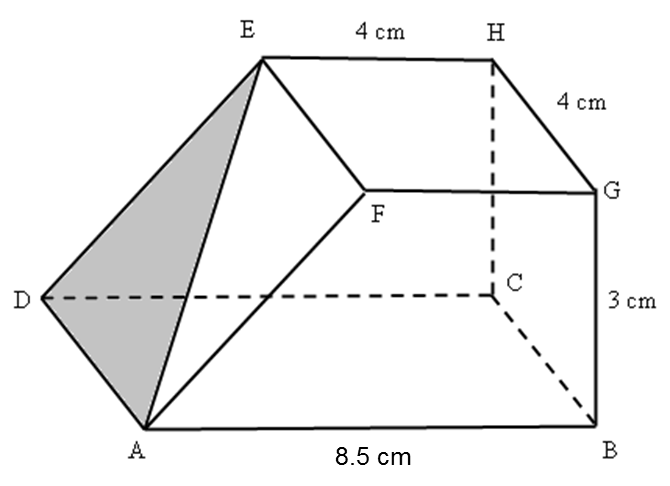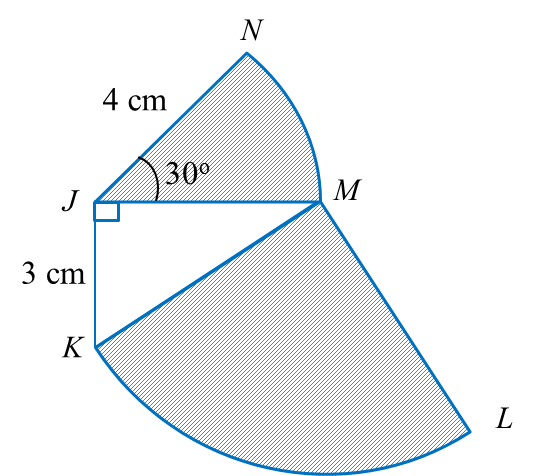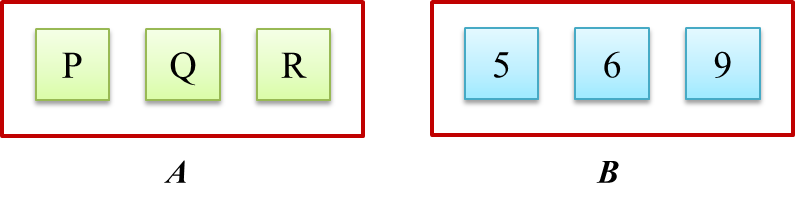# SPM Mathematics (Model Test Paper)

SPM Mathematics (Model Test Paper)

Section A
(52 marks)
Answer all questions in this section.
1. The Venn diagram in the answer space shows sets E, and G such that the universal set, $\xi =E\cup F\cup G.$
On the diagrams in the answer space, shade
$\begin{array}{l}\left(a\right)\text{}E\text{}\text{'}\cap G\text{'}\\ \left(b\right)\text{}\left(E\cap F\right)\text{'}\text{}\cup G\text{'}\end{array}$
[3 marks]2.   Calculate the value of x and of that satisfy the following simultaneous linear equations:
$\begin{array}{l}x+6y=12\\ \frac{2}{3}x+2y=6\end{array}$
[4 marks]

3.Diagram 1

Diagram 1 shows a square ABCD and a right-angled triangle PQR with equal area.
Based on the information, find the value of x.
Hence, find the perimeter, in cm, of the square ABCD. [4 marks]

4 (a) For each of the following statements, determine whether the statement is true or  false.
(i) 35 is multiple of  3 and 5
(ii)   7 is a factor of 42 or 16 is a multiple of 6.
(b)   Write down two implications based on the following sentence:
p3 = –8 if and only if p = –2
(c) Make a general conclusion by induction for a list of numbers 2, 11, 26, 47, …….   which  follows the following pattern.
2 = 3(1)2– 1
11 = 3(2)2– 1
26 = 3(3)2– 1
47 = 3(4)2– 1
……………
[6 marks]

5.   Diagram 2 in the answer space shows a right prism. Trapezium ABGF  is the uniform cross section of the prism.
(a) On Diagram 2, mark the angle between the plane ADE and the plane ADCB.
(b) Calculate the angle between the plane ADE and the plane ADCB.
[3 marks]
(a)Diagram 2

6.   Diagram 3 shows a right cylinder with a diameter of ( y  + 4 ) cm.Given the volume of the cylinder is 269.5 cm3 and by using  find the value of it’s radius.
[4 marks]

7.   Diagram 4 shows a straight line ST and a straight line PQ drawn on a Cartesian plane.  ST is  parallel to PQ. Given that equation of the straight line ST is 2y = 8x + 3.Diagram 4

Find,
(a)  the equation of the straight line PQ,
(b)  the x-intercept of the straight line PQ.
[ 5 marks ]

8.   Diagram 5, shows a quadrant KLM with centre  M and sector JMN with centre J.Diagram 5

Using $\pi =\frac{22}{7}$ , calculate
(a) the perimeter, in cm, of the whole diagram,
(b) the area, in cm2, of the shaded region.
[6 marks]

9. Diagram 6 shows the speed-time graph for the movement of two particles, J and K, for a period of t s. The graph ABCD represents the movement of J and the graph AE represents the movement of K. Both particles start at the same point and move along the same route.Diagram 6
(a) State the uniform speed, in ms-1, of particle J.
(b)  Calculate the rate of change of speed, in ms-2, of particle J for the first 13 s.
(c) At t s, the difference between the distance travelled by J and K is 169 m. Calculate the value of t.
[ 6 marks ]

10.   Diagram 7.1 shows three cards labelled with letters in bag A and three numbered cards in bag B.Diagram 7.1

A card is picked at random from bag A and then a card is picked at random from bag B.
(a) Diagram 7.2 in the answer space shows the incomplete possible outcomes of the  event. Complete the possible outcomes in Diagram 7.2.
(b) Using the complete possible outcomes in 10(a), find the probability that
(i) a card labelled Q and the card with odd number are picked,
(ii) a card labelled P or the card with a number which is multiple of 3 are picked.
[5 marks]
(a)Diagram 7.2

11.   It is given that matrix M is a 2 × 2 matrix such that

(a) Find matrix M.
(b) Write the following simultaneous linear equations as matrix equations:
–2x + y = 10
x + 3y= 9
Hence, using matrix method, calculate the value of x and of y. [6 marks]